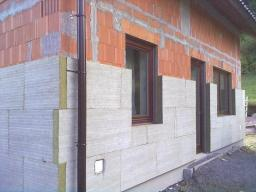# Insulation

Two workers insulate the house for five days. After the first day of working together, only one worker continue to cover the house. How many days will the house be insulated if every worker has the same work tempo?

x =  9 d

### Step-by-step explanation:

2·1 + 1·(x-1) = 2·5

x = 9

x = 9/1 = 9

x = 9

Our simple equation calculator calculates it.Did you find an error or inaccuracy? Feel free to write us. Thank you!

Tips for related online calculators
Do you have a linear equation or system of equations and looking for its solution? Or do you have a quadratic equation?
Do you want to convert time units like minutes to seconds?